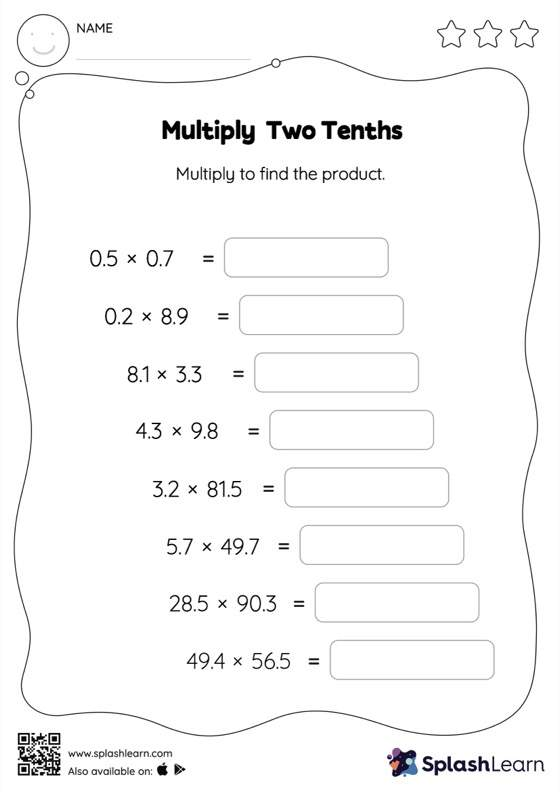# Multiply Two Tenths: Horizontal Multiplication Worksheet

Home > Multiply Two Tenths: Horizontal MultiplicationWhen a tenth is multiplied by another tenth, the product will have two decimal digits. Students first multiply the numbers ignoring the decimal points, and then add the decimal point two places from the right in the product. They practice this concept extensively in multiply two tenths worksheet. As the worksheet uses a horizontal format, it allows for more creativity when it comes to how the student solves the problem. In contrast, in the vertical format, students usually employ the standard method to solve.